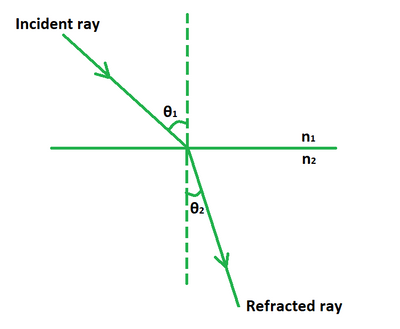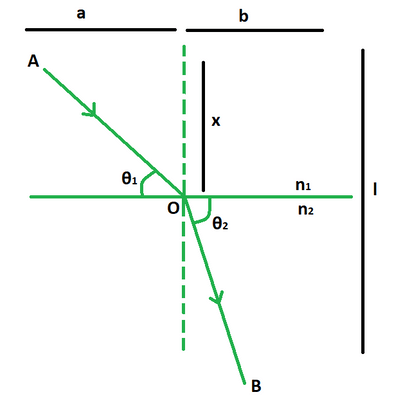# Snell’s Law Formula

• Last Updated : 24 May, 2022

Snell’s law explains the relationship between the angle of refraction, incidence, and the refractive law of indices for the specified media such as light, glass, and air. It asserts that the ratio of the sines of the angle of incidence 1 and angle of refraction 2 for a given set of media is equal to the ratio of phase velocities (v1/v2) in the two media, or equivalently, the refractive indices (n2/n1). When light moves from one medium to another, it undergoes either bending or refraction. The angle of bending is determined by using Snell’s law.### Snell’s Law Formula

n1 sin θ1 = n2 sin θ2 = μ

Where,

• n1 is the initial media,
• θ1 is the angle of incidence,
• n2 is the final media,
• θ2 is the angle of refraction,
• μ is the refractive index.

DerivationSuppose an incident ray OA undergoes refraction from refractive index n1 to that of n2.

The phase velocities of light ray in medium 1 and 2 are,

v1 = c/n1, v2 = c/n2 ⇢ (1)

The time taken (by incident ray) from A to O is,

t1 = √(x2 + a2)/v1

The time taken (by refracted ray) from O to B is,

t2 = √(b2 + (l – x)2)/v2

To minimize the total time, we must equate its differential to zero.

dt/dx = 0

dt1/dx + dt2/dx = 0

x/v1√(x2 + a2) – (l – x)/v2√(b2 + (l – x)2) = 0

sin θ1/v1 = sin θ2/v2

From (1) we get,

sin θ1/(c/n1) = sin θ2/(c/n2)

n1 sin θ1 = n2 sin θ2

This derives the formula for Snell’s law.

### Sample problems

Problem 1: Calculate the refractive index if angle of incidence is 15° and angle of refraction is 20°.

Solution:

We have,

θ1 = 15°

θ2 = 20°

Using the formula we get,

μ = sin θ1/sin θ2

= sin 15°/sin 20°

= 0.2588/0.342

= 0.75

Problem 2: Calculate the refractive index if angle of incidence is 25° and angle of refraction is 30°.

Solution:

We have,

θ1 = 25°

θ2 = 30°

Using the formula we get,

μ = sin θ1/sin θ2

= sin 25°/sin 30°

= 0.422/0.5

= 0.211

Problem 3: Calculate the refractive index if angle of incidence is 23° and angle of refraction is 40°.

Solution:

We have,

θ1 = 23°

θ2 = 40°

Using the formula we get,

μ = sin θ1/sin θ2

= sin 23°/sin 40°

= 0.39/0.64

= 0.60

Problem 4: Calculate the angle of incidence if refractive index is 0.56 and angle of refraction is 50°.

Solution:

We have,

μ = 0.56

θ2 = 50°

Using the formula we get,

μ = sin θ1/sin θ2

=> sin θ1 = μ sin θ2

=> sin θ1 = 0.56 (sin 50°)

=> sin θ1 = 0.42

=> θ1 = 24.83°

Problem 5: Calculate the angle of incidence if refractive index is 0.23 and angle of refraction is 32°.

Solution:

We have,

μ = 0.23

θ2 = 32°

Using the formula we get,

μ = sin θ1/sin θ2

=> sin θ1 = μ sin θ2

=> sin θ1 = 0.23 (sin 32°)

=> sin θ1 = 0.126

=> θ1 = 7°

Problem 6: Calculate the angle of refraction if refractive index is 0.78 and angle of incidence is 45°.

Solution:

We have,

μ = 0.78

θ1 = 45°

Using the formula we get,

μ = sin θ1/sin θ2

=> sin θ2 = sin θ1

=> sin θ2 = sin 45°/0.78

=> sin θ2 = 0.906

=> θ2 = 64°

Problem 7: Calculate the angle of refraction if refractive index is 0.65 and angle of incidence is 38°.

Solution:

We have,

μ = 0.65

θ1 = 38°

Using the formula we get,

μ = sin θ1/sin θ2

=> sin θ2 = sin θ1

=> sin θ2 = sin 38°/0.65

=> sin θ2 = 0.947

=> θ2 = 71°

My Personal Notes arrow_drop_up
Recommended Articles
Page :